# Recursive Procedure for Reversing a Linked List

## Recursive Procedure for Reversing a Linked List in C Language

In this article, I am going to discuss the Recursive Procedure for Reversing a Linked List in C Language with Examples. Please read our previous article, where we discussed How to Reverse a Linked List by Reversing Links in C Language with Examples.

##### Recursive Procedure for Reversing a Linked List in C

Let us look at the recursive procedure for reversing a linked list. Already we have learned recursion in many topics so we know that recursion will have two phases. One is the calling phase and another one is returning phase.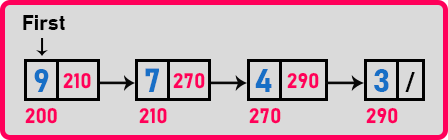Now let us decide, shall we reverse the link of each node while calling or while returning. We should do it in the returning time. If we are doing it while calling then how we can get the address of the next node?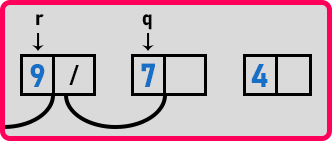But while returning we can make a node point on the previous node. Let us see how we can do this?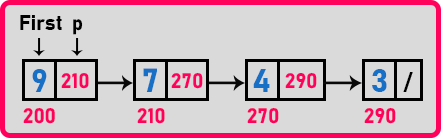Let us take a pointer ‘p’ pointing on the first node and it will move on to the next node in every function call. So, after all the function calls ‘p’ will become null.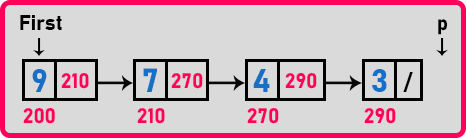Now, ‘p’ is null after all the recursive calls and while returning from the function call, ‘p’ will point to the last node and in order to make the last node point on the previous node, we need one tail pointer also to store the address of the previous node. Now let us start again with two pointers as,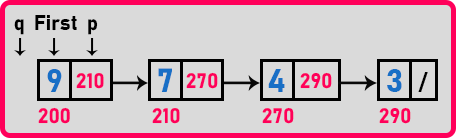Here ‘q’ is pointing to null and ‘p’ is pointing to the first node i.e. ‘9’. So, after all the recursive calls ‘q’ will be pointing to the last node and ‘p’ will be pointing to null.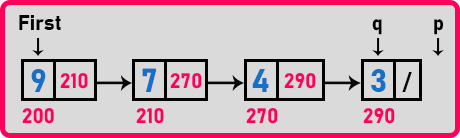While returning time ‘q’ will point to node ‘4’ and ‘p’ will point to node ‘3’.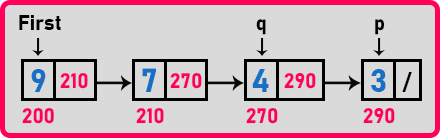Here we will modify ‘p’ as ‘p->next = q’. In this way, we will reverse all the nodes of the linked list. Now let us write a function for revering links recursively,

##### Recursive Procedure for Reversing a Linked List Pseudo Code:

void Reverse(Node *q, Node *p){
if(p != NULL){
Reverse(p, p->next);
p->next = q;
}
else
first = q;
}

##### Program for Recursive Procedure for Reversing a Linked List using C Language:

Let us see the program which uses a recursive function to reverse a linked list.

```#include <stdio.h>
#include <stdlib.h>

struct Node
{
int data;
struct Node *next;
} *first = NULL;

void Create(int A[], int n)
{
int i;
struct Node *t, *last;
first = (struct Node *) malloc(sizeof(struct Node));
first->data = A;
first->next = NULL;
last = first;

for (i = 1; i < n; i++)
{
t = (struct Node *) malloc(sizeof(struct Node));
t->data = A[i];
t->next = NULL;
last->next = t;
last = t;
}
}

void Display(struct Node *p)
{
while (p != NULL)
{
printf ("%d ", p->data);
p = p->next;
}
}

void RReverse(struct Node * q, struct Node * p)
{
if (p != NULL)
{
RReverse (p, p->next);
p->next = q;
}
else
first = q;
}

int main()
{
int A[] = { 3, 4, 9, 10, 13, 16, 19 };
Create (A, 5);
struct Node *q = NULL, *p = first;

printf ("Before Reverse: \n");
Display (first);
RReverse (q, p);

printf ("\nAfter Reverse: \n");
Display (first);
return 0;
}
```
###### Output: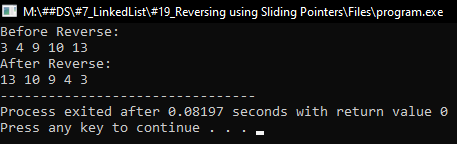In the next article, I am going to discuss How to Concatenate Two Linked Lists in C Language with Examples. Here, in this article, I try to explain the Recursive Procedure for Reversing a Linked List in C Language with Examples and I hope you enjoy this Recursive Procedure for Reversing a Linked List in C Language with Examples article.